# Android JNI 編程 - C語言基礎知識 (一)

## 基礎環境搭建

```# CLion remote docker environment (How to build docker container, run and stop it)

# ssh credentials (test user):

FROM centos:7

RUN sed -e 's|^mirrorlist=|#mirrorlist=|g' \ -e 's|^#baseurl=http://mirror.centos.org|baseurl=https://mirrors.tuna.tsinghua.edu.cn|g' \ -i.bak \ /etc/yum.repos.d/CentOS-*.repo

RUN yum -y update \ && yum -y install openssh-server \ make \ autoconf \ automake \ locales-all \ dos2unix \ ninja-build \ build-essential \ gcc \ gcc-c++ \ gdb \ clang \ cmake \ rsync \ tar \ python \ && yum clean all

RUN ssh-keygen -A

RUN ( \ echo 'LogLevel DEBUG2'; \ echo 'PermitRootLogin yes'; \ echo 'PasswordAuthentication yes'; \ echo 'Subsystem sftp /usr/libexec/openssh/sftp-server'; \ ) > /etc/ssh/sshd_config_test_clion

CMD ["/usr/sbin/sshd", "-D", "-e", "-f", "/etc/ssh/sshd_config_test_clion"] ```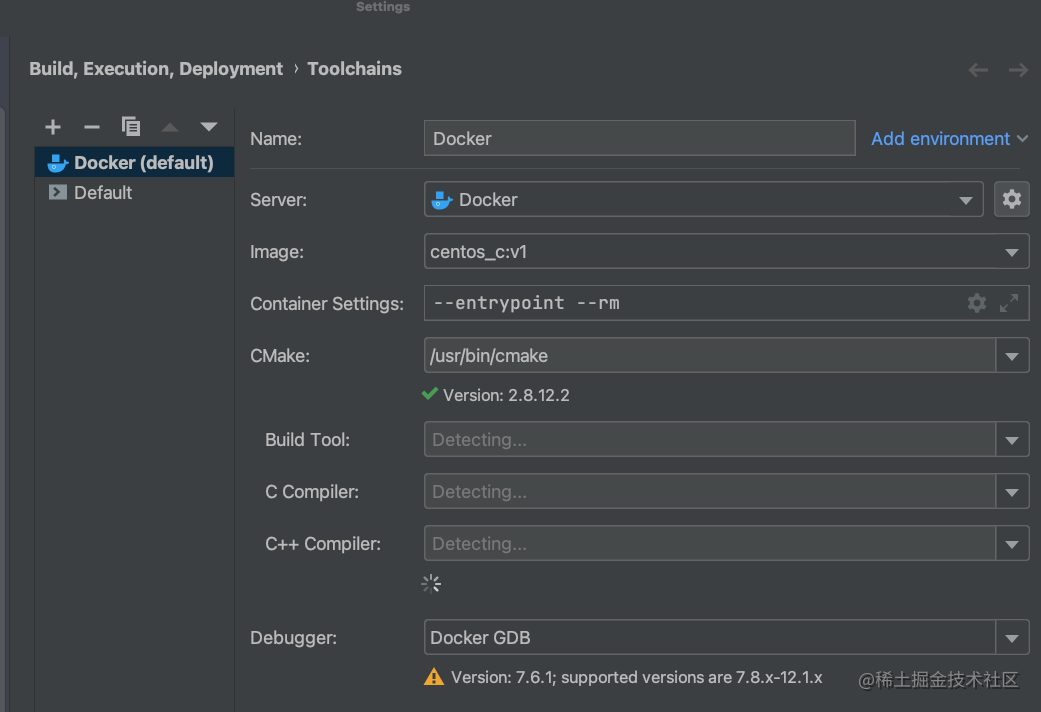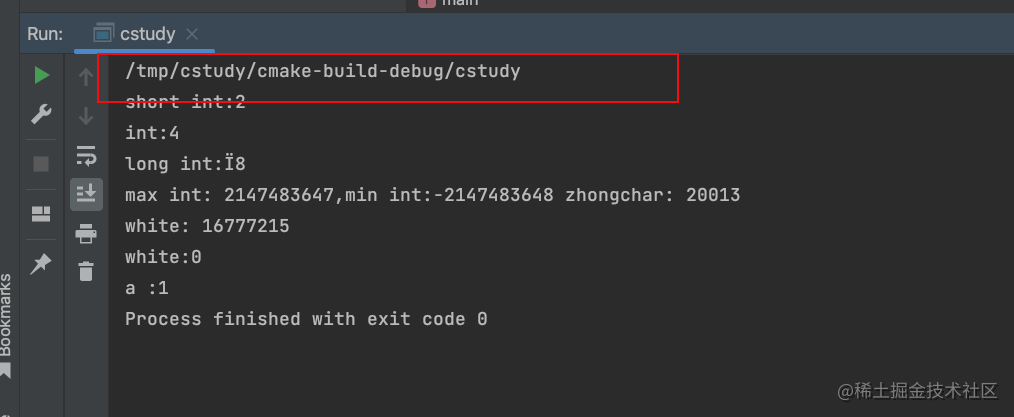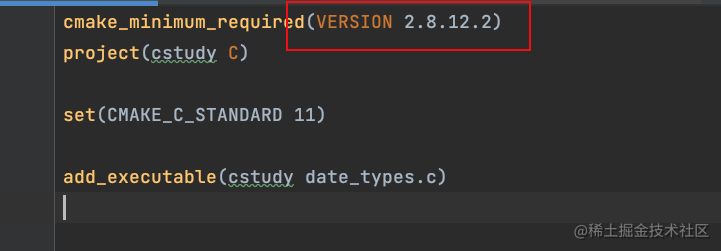``` cmake_minimum_required(VERSION 2.8.12.2) project(cstudy C)

set(CMAKE_C_STANDARD 11)

```

# include

void HandleVarargs(int arg_count,...) { // 用於獲取變長參數 va_list args; // 開始遍歷 va_start(args, arg_count); int j; for ( j = 0; j < arg_count; ++j) { // 取出對應參數 int arg = va_arg(args, int); printf("%d: %d \n", j, arg); } // 結束遍歷 va_end(args); }

int main() { HandleVarargs(4,1,2,5,6); return 0; } ``` 在c中 寫個變長參數的函數 真的累。。。 還要寫這種模版代碼

## 宏

### 頭文件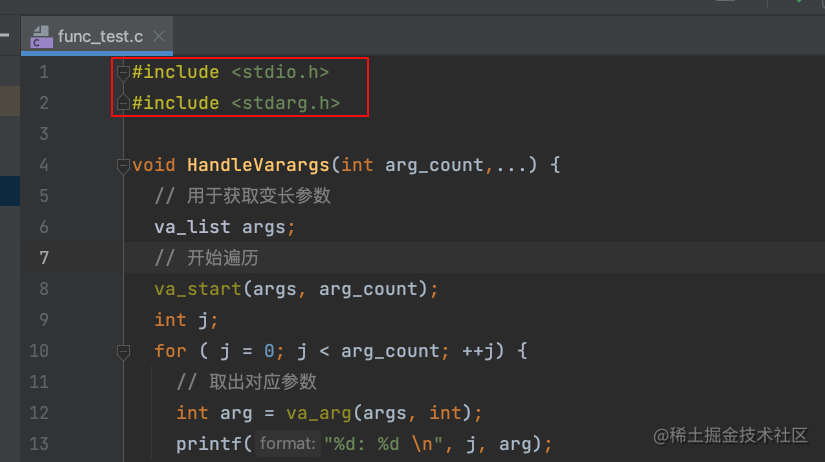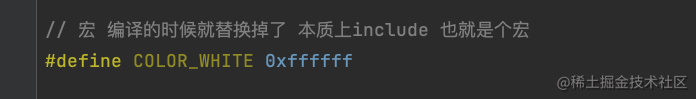### 自己寫頭文件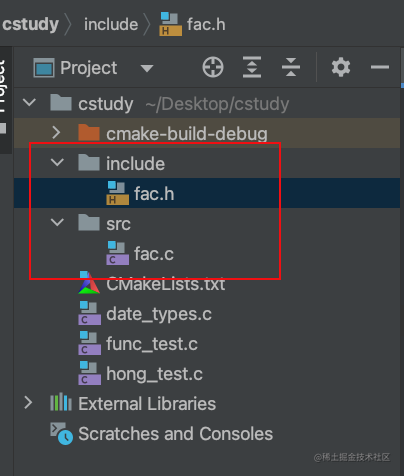``` // // Created by 吳越 on 2023/2/18. //

# define CSTUDY_INCLUDE_FAC_H_

int sum(int x,int y);

# endif //CSTUDY_INCLUDE_FAC_H_

```

``` // // Created by 吳越 on 2023/2/18. //

# include "../include/fac.h"

int sum(int x,int y){ return x+y; } ```

`add_executable(cstudy3 hong_test.c src/fac.c)`

```

# include "include/fac.h"

int main() { printf("sum: %d \n", sum(3, 5)); return 0; } ``` 就這麼簡單！

### <> 和 ““ 的區別

`include_directories("include")`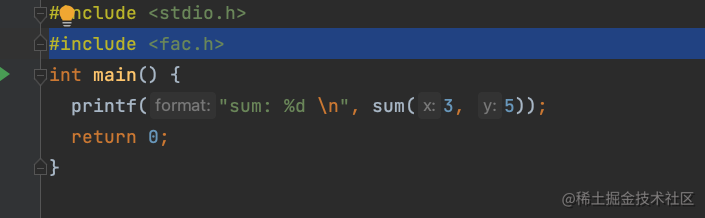```

# define MAX(a, b) a>b?a:b

int main() { printf("max : %d \n", MAX(1, 3)); printf("max : %d \n", MAX(1, MAX(4,3)));

return 0; } ``` 看下執行結果：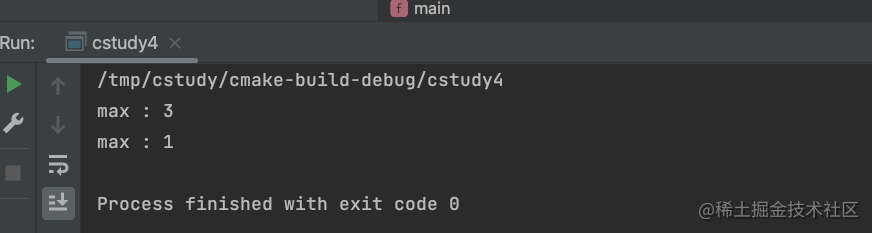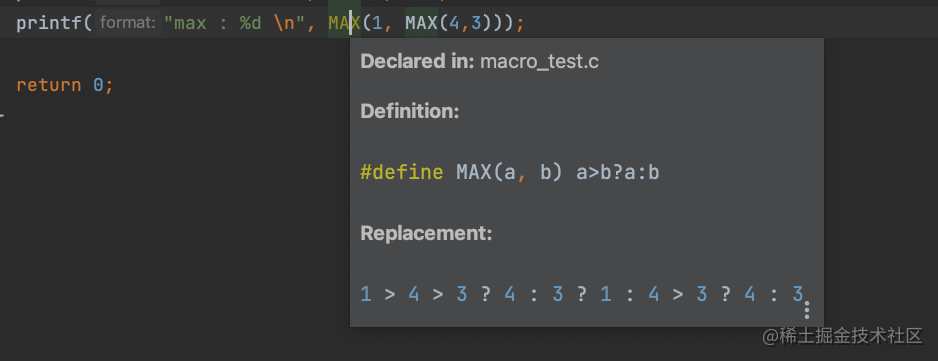```

# define MAX(a, b) (a) >(b) ? (a) :(b)

```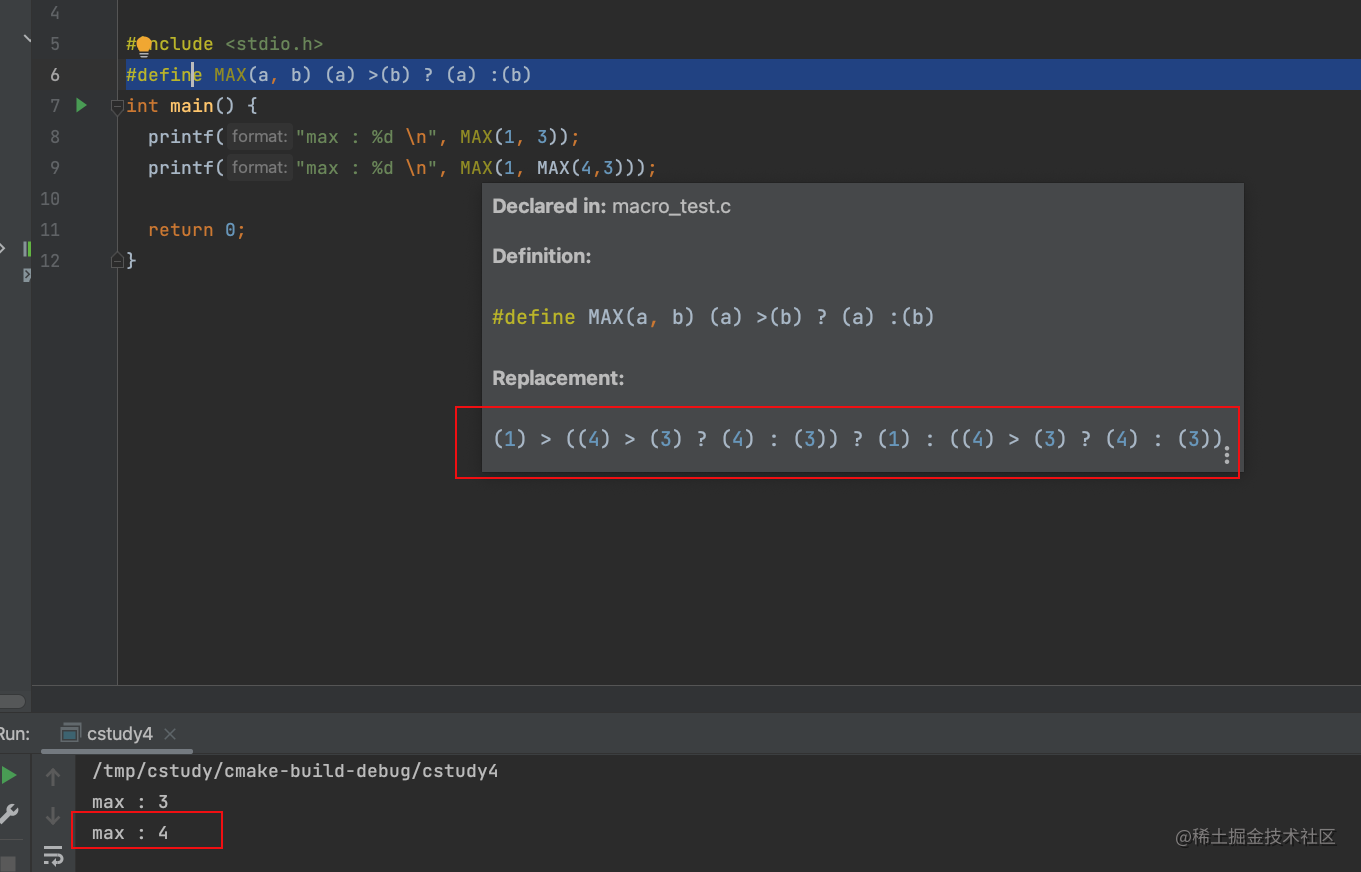```

# define MAX(a, b) (a) >(b) ? (a) :(b)

#define is_hex_char(c) \ ((c)>='0'&& (c)<='9') || \ ((c)>='a' && (c)<='f') int main() { printf("max : %d \n", MAX(1, 3)); printf("max : %d \n", MAX(1, MAX(4, 3))); printf("is hex : %d \n", is_hex_char('a')); return 0; } ```

### 條件編譯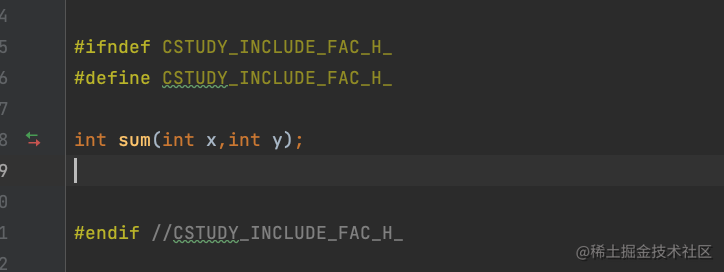```

void dump(){

# ifdef DEBUG

printf("debug info");

# endif

}

int main(){ dump(); return 0; } ``` 然後在cmake中寫一下：

```add_executable(cstudy5 macro_test2.c) target_compile_definitions(cstudy5 PUBLIC DEBUG)```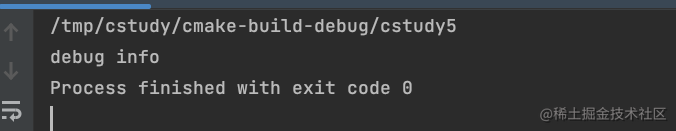```

# define PRINTFLINE(format, ...) printf(format"\n",##VA_ARGS)

void Printf(const char *format, ...) { va_list args; va_start(args, format); vprintf(format, args); printf("\n"); va_end(args); }

int main() { Printf("hello"); PRINTFLINE("world"); return 0; } ```

```

# define PRINT_INT(value) PRINTFLINE(#value": %d",value)

```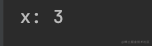```

# define PRINTFLINE(format, ...) printf("("FILE":%d) %s : "format"\n",LINE, FUNCTION, ##VA_ARGS)

```

## 字符串

### 字符串基本知識

c 語言中的字符串 必須以 null 也就是\0 為結尾

```

# include

int main(){ char string="wuyue"; char string2="wuyue"; PRINTFLINE("string: %s",string); PRINTFLINE("string2: %s",string2);

return 0; } ``` 來看下執行結果：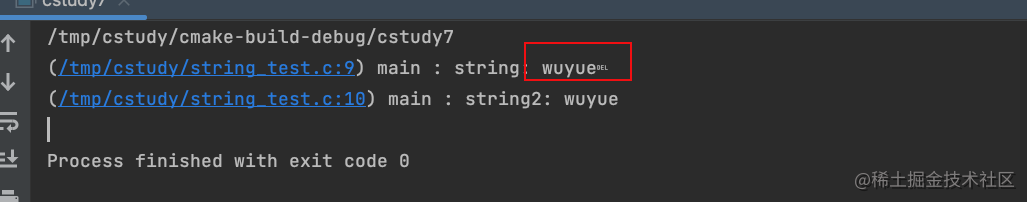## 指針

### 指針的只讀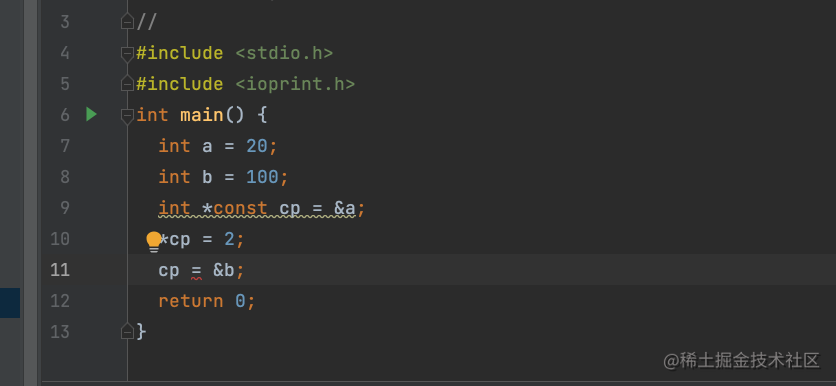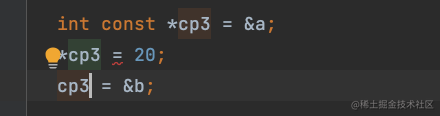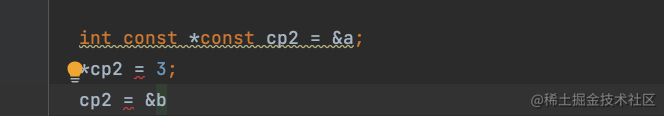const 如果修飾的是指針，則地址不能修改 const 如果修飾的是值，則值不能修改

```

# include

int main() {

int array = {0}; int pa= array; pa=2; (pa++)=3; (pa+2)=4; PRINT_INT_ARRAY(array,4); return 0; } ```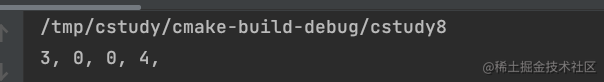### 指針參數作為返回值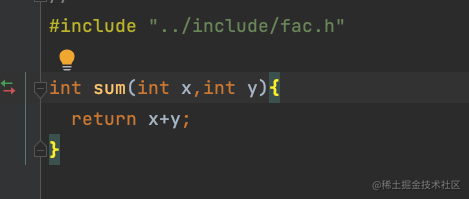```void sum2(int x, int y, int *result) { *result = x + y; }```

```

# include

int main() { int size = 10; int *array = malloc(sizeof(int) * size); int i; for (i = 0; i < size; ++i) { array[i]=i; } PRINT_INT_ARRAY(array,size) free(array); return 0;

} ```

``` void InitIntArray(int *a, int size, int defaultValue) { a = malloc(sizeof(int) * size); int i; for (i = 0; i < size; ++i) { a[i] = defaultValue; } }

int main() { int size = 10; int *a; InitIntArray(a,size,0); PRINT_INT_ARRAY(a, size) free(a); return 0; } ```

int main() { int size = 10; int *a; InitIntArray2(&a,size,0); PRINT_INT_ARRAY(a, size) free(a); return 0; } ```

```// 自動初始化 int *b = calloc(size, sizeof(int)); // 在之前的基礎上，重新開闢一段空間，等於是擴大了 b = realloc(b, size * 2); if (b != NULL) { PRINTFLINE("分配成功"); PRINT_INT_ARRAY(b, size); } else{ PRINTFLINE("分配失敗"); } return 0;```

### 函數指針

```void (*func)(int **aparams, int size, int defaultValue) = &InitIntArray2; size = 30; func(&a, size, 30); PRINT_INT_ARRAY(a, size);```

``` // f1是一個函數，這個函數的返回值 是一個int * 指針 int *f1(int,double );

// f2是一個函數指針，指向一個 參數為int，double 返回值是int的 函數 int (*f2) (int,double );

// f3和f2 一樣，只不過返回值 是一個 int * 的函數 int (f3) (int,double );

// 函數的指針可以定義數組，但是函數不能定義數組 int (*f5[]) (int,double); ```

```// 定義這個函數指針的別名 typedef int (*Func)(int, int); int Add(int x, int y) { return x + y; }``` 然後去調用他

```Func func_1 = &Add; PRINTFLINE("result : %d" ,func_1(3, 4));```